Disclaimer: This is an example of a student written essay.

Any scientific information contained within this essay should not be treated as fact, this content is to be used for educational purposes only and may contain factual inaccuracies or be out of date.

# First-principles Study of Spin Transport in Si-C Nanotube

 ✅ Paper Type: Free Essay ✅ Subject: Sciences ✅ Wordcount: 3379 words ✅ Published: 8th Aug 2018

### First-principles study of spin transport in Si-C nanotube with ferromagnetic contacts

• Surendra Jalu

Abstract — We perform first-principles calculations of spin-dependent quantum transport in a two-probe device, viz. Fe-SiCNT-Fe device in which (8,0) Si-C nanotube is sandwiched between two Fe electrodes. Substantial tunnel magnetoresistance and perfect spin filtration effect are obtained. The zero bias tunnel magnetoresistance is found to be several hundred percent, it reduces monotonically till the bias voltage 0.4 V, sees marginal increase at the bias of 0.6 V, and eventually goes to nearly zero after the bias voltage of about 1 V. The spin injection factor is 100% at the zero bias, it reduces sharply to 57% at the bias of 0.4 V, and remains reasonably high, in the range of 60%-75%, after the bias voltage of 0.6 V when the magnetic configurations of two electrodes are parallel. The spin-dependent non-equilibrium transport features can be understood by analyzing microscopic details of the transmission coeï¬ƒcients.

Keywords — First-principles; Tunnel magnetoresistance(TMR); Spin injection; One-dimensional; Spin filter

## I. Introduction

Si-C crystals have unique properties, including thermal stability, chemical inertness, high thermal conductivity, and others. These properties make Si-C materials an appropriate candidate for fabricating electronic devices operating in high power, high temperature environments. Recently synthesized Si-C nanometer materials, such as nanorods, nanowires and nanotubes, have attracted tremendous interest (1-4). These materials obviously broadened the application range of the Si-C crystals, especially in fabricating nanometer gas sensors, electronic devices, field emitters and other novel devices.

If you need assistance with writing your essay, our professional essay writing service is here to help!

Silicon carbide nanotubes have a great potential for application in chemical and biological sensors (Taguchia et al 2005a; Xie et al 2007). Recently, scientists have suggested to make silicon carbide nanotubes (SiCNTs) as a possible alternative to carbon nanotubes (CNTs) for field emitting applications (Taguchia et al 2005b; Alfieri and Kimoto 2010), which has boosted the interest for SiCNTs and several studies on the electronic properties of SiCNTs can be found in the literature (Mavrandonakis et al 2003; Mpourmpakis et al 2006; Larina et al 2007; Muralidharan et al 2007; Wu and Guo 2007; Lakshmi et al 2008; Moradian et al 2008; Raidongia et al 2008; Voggu et al 2008; Wu et al 2008; Manna and Pati 2010; Zheng et al 2010). It is well known that CNTs undergo a semiconductor to metal transition (SMT) (Ren et al 2009) or metal to semiconductor transition (MST) (Lu et al 2003) when deformed. Therefore, it is of interest to understand how the semiconducting (8,0) SiCNT would behave when it is brought in contact with ferromagnetic electrodes.

On account of important applications in magnetoresistive random access memory , programmable logic elements  and magnetic sensors, the giant magnetoresistance (GMR) and tunnel magnetoresistance (TMR) effects in systems with spin-polarized transport are at the heart of spintronics  and attract many theoretical and experimental investigations. A basic magnetic tunnel junction (MTJ) device consists of tunnel barrier separating two ferromagnetic (FM) layers which play the role of device leads. During a tunneling process, the spin-up and spin-down electrons from the metal layers traverse the nonmagnetic tunnel barrier with different Fermi wave function due to electronic structure of the ferromagnetic material. The electrical resistance of MTJ is therefore sensitive to the relative orientation of the magnetic configuration of the electrodes, resulting to a TMR . Hence, the MTJs behave as spin valves [4–8]. It is possible to control the orientation of magnetic moments by imposing an external magnetic field in experiments [9,10]. It is important to note that, MgO-based MTJ has progressed at a rapid pace in recent years and produced the highest measured TMR at room temperature.

When the electrodes and the tunnel barrier are all one-dimensional (1D) materials such as nanowires or nanotubes, it is experimentally feasible to achieve MTJ at the 1D scale. It is worth mentioning that Wang et al.  construct a 1D TMJ using Fe-doped carbon nanotubes and theoretically investigate its spin-dependent transport properties. What is a pity that, the zero bias magnetoresistance ratio is only ∼ 40%, which may restrict the spintronics applications in nanodevices where the large magnetoresistance ratio is desirable. Here, we theoretically investigate the spin-polarized transport of a 1D MTJ constructed by (8,0) SiCNT in contact with FM(Fe) electrodes. It is observed that (8,0) SiCNTs are semiconducting and we believe that it can be used as a tunnel barrier for 1D or molecular-scaled MTJ. In our work, We have chosen (8,0) SiCNT as the tunnel barrier and two semi-infinite FM materials, namely Fe, as electrodes. Significant TMR and perfect spin filtration effect are obtained according to the first-principles calculations of spin-polarized quantum transport by adopting non-equilibrium Green’s function method combined with density-functional theory. TMR has already led to the construction of the present generation of magnetic data storage devices. However, in order to reach storage densities of the order of Terabit/inch2, a substantial down-scaling of the read/write devices is extensively expected. Such 1D MTJ and spin filter with diameter less than 1 nm may realize the storage densities of the order of Terabit/inch2 and hold promise for extensive spintronics applications of nanodevices.

## II. Model and method

Our investigation is based on a recently developed self-consistent first-principles technique which combines the Keldysh non-equilibrium Green’s-function formalism (NEGF) with a self-consistent density-functional theory (DFT). The package we use is the Atomistix Toolkit , which incorporates the NEGF technique into the well tested SIESTA method  to realize the simulation of electrical or spin-polarized quantum transport in the molecular conductors under non-equilibrium situations. In the calculation, the local density approximation (LDA) in the form of the Perdew and Zunger  (perdew burke ernzerhof) exchange-correlation functional is used. Only valence electrons are self-consistently calculated, and the atomic cores are described by standard norm conserving pseudopotential . The valence wave functions are expanded by the localized numerical (pseudo) atom orbitals . The k-points sampling is 3, 3, and 200 in the x, y, and z direction, which has been proven to be enough to give the converged results. The convergence criterion for the Hamiltonian, charge density, and band-structure energy is 10-5 via the mixture of the Hamiltonian. The positions of C and Fe atoms at the interface of the device are relaxed until the force tolerance 0.05 eV/Å is achieved. The spin-current (spin-polarized charge current) is calculated  as

I↑(↓) = e/h ∫ T↑(↓)(E, Vb) [F(E − μL) – F(E − µR)] dE [R]

Where F is the Fermi–Dirac distribution, μL and μR are the chemical potentials of left-electrode (L) and right-electrode (R) respectively, T↑(↓)(E, Vb) is the transmission coeï¬ƒcient for spin channel ( ↑ , ↓ ) at the energy E and bias voltage Vb.

While building SiCNT, it is utterly important to use optimum bond-length between Si and C atoms to obtain correct results which in our case, as mentioned in various literatures, is 1.78 Å. We couldn’t find the optimum bond-length between C and Fe atoms in literature but we were able to optimize a geometry that consisted of only a few atoms of Fe and C and this way we found the optimum bond-length between Fe and C atoms to be 2.04 Å (Fig. 4 (b)). The whole system (Fig. R) is divided into three parts from left to right in practical theoretical simulations: the left electrode, the central scattering region, and the right electrode. The left electrode and right electrode are exactly the same and are made of Fe atoms. Si-C nanotube acts as central scattering region between two electrodes. The whole structure contains 328 atoms (192 Fe, 72 C, 64 Si).

##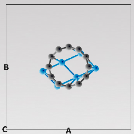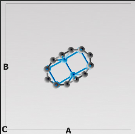4 (a) 4 (b)

Fig. 4(a) Fe-C bond-lengths in non-optimized geometry 4(b) Fe-C bond lengths in optimized geometry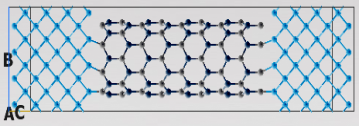Fig. 4(c) Fe-SiCNT-Fe device

## III. results and discussions

Fig. 2(a) and (b) plot the current–voltage (I–V) characteristics for the parallel magnetization configuration (PC) and the antiparallel magnetization configuration (APC) of the two electrodes, respectively. In the case of PC, the spin-up current (I↑) are always much larger than the spin-up current (I↓). The I↑ increases steeply with bias voltage especially after the bias of 0.8 V while on the other hand I↓ is almost negligibly small compared to I↑ at almost all bias voltages. The total equilibrium conductance of 0.0022G0 is found at zero bias, where G0 is the conductance quanta, G0 = 2e2/h. In the case of APC, I↑ and I↓ have almost the same values at all bias voltages. The total equilibrium conductance of 0.0095G0 is found at zero bias.

From the I–V curves, we infer a TMR ratio using the common definition: TMR = (IAPC − IPC)/IAPC, where IPC and IAPC are the total currents in PC and APC, respectively. At Vb = 0 when all currents vanish, we calculate TMR using equilibrium conductance. Fig. 2(c) shows the TMR, where the RTMR ∼ 322% at zero bias is obtained, which indicate in the perfect tunneling magnetoresistance effect. It sharply decreases to ~ 80% at the bias voltage of 0.4 V, sees slight increase at the bias voltage of 0.6 V and gets reduced to almost zero after the bias voltage of 1 V. The TMR decrease with bias voltages because IPC increases at higher rate with bias voltages than IAPC. It is possible for TMR to assume negative value but in our case no such behavior is observed. The device structure presented here indeed has substantial TMR and could be utilized for spintronics applications.

The spin-injection factor η which is defined by spin currents: η = mod(I↑ – I↓) / (I↑ + I↓) are plotted in Fig. 2(d). At the bias voltage of 0.0 V, the current is 0 A and hence we use the equilibrium conductance represent current. For the case of PC, η is 99% at the zero bias, it monotonically reduces to 57%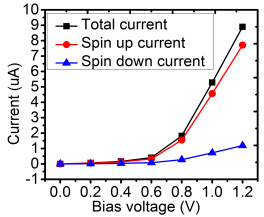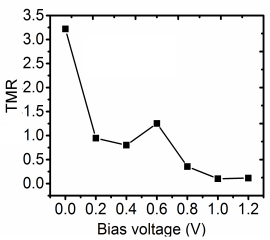at the bias voltage of 0.4 and it increases and remains fairly within large values once the bias voltage goes beyond 0.4 V. The spin-injection factor η is as large as ~73% at the bias of 1.2 V and may even improve at higher bias voltages. For the case of APC, the η is meager ~49% at the zero bias. It’s dramatic that it reaches ~85% at the bias voltage of 0.2 V but then it keeps reducing with increase in bias voltages and attains very negligible values at the bias of about 1 V to 1.2 V. From Fig.R it is observed that in the case of PC the spin filtration effect manifests itself very clearly when the bias is above 0.4 V while in the case of APC no such effect is seen. The source of this behavior maybe originates from the quantum size effect which results in the quantized subbands in the cross section of 1D electrode. Detailed analysis is in the below. Spin injection into semiconductors has been measured experimentally using the optical techniques [26,27]. Such 1D TMJ controlled spin injection into semiconductors should occur in devices that are smaller than a spin relaxation length in extent and have resistance that is limited by the insulating BNNT.

Our academic experts are ready and waiting to assist with any writing project you may have. From simple essay plans, through to full dissertations, you can guarantee we have a service perfectly matched to your needs.

The voltage dependent of spin current, TMR ratio and spin-injection factor can be understood from the behavior of the transmission coeï¬ƒcients [ T (E, Vb) ] , since the current is essentially given by the energy integral of the T (E, Vb) over the bias window [see Eq. (1)]. The T (E, Vb) for several bias are shown in Fig. 3, where the (a) and (b) are spin-up and spin- down channel of PC setup respectively and the (c) and (d) are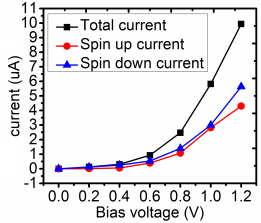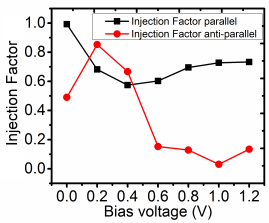spin-up and spin-down channel of APC setup respectively. For both PC and APC setup, these bias-depended T(E,Vb) have several sharp peaks especially after the bias of 0.35 V, due to quantized subbands in the cross section of the 1D electrodes, already mentioned above. The spin up channel contribute to the T(E,Vb) above the Fermi level and spin-down channel contribute both above and below the Fermi level. Refer to the notation from molecular transport theory  we can deduce that the spin-up channel conductance is through the lowest unoccupied molecular orbital (LUMO) while the spin-down channel conductance is through both the highest occupied molecular orbital (HOMO) and LUMO of pristine (6,0) BNNT in scattering region.

From Fig. 3(a), one can see that the T (E, Vb) shifts to high energy but roughly maintains its shape when bias increases. The T (E, Vb) are all almost absolutely inhibited in spin-up channels around Fermi level, while in the spin-down channel the T (E, Vb) is smooth and several orders of magnitude larger than in spin-up channel around Fermi level. This is why the I↓ linearly increases while the I↑ is inhibited under the bias of 0.75 V, already discussed above for Fig. 2(a). As the bias increases, the electrochemical potential in the left electrode (μL) is shifted down, and that in the right electrode (μR) is shifted up. Hence, the bands in the two electrodes are shifted correspondingly [29–32]. When the bias increase beyond 0.75 V, a little resonance states A1 are induced around Fermi level showed in Fig. 3(a) for the bias of 0.75, 0.95 and 1.15 V, which originate from the alignment of the LUMO of left electrode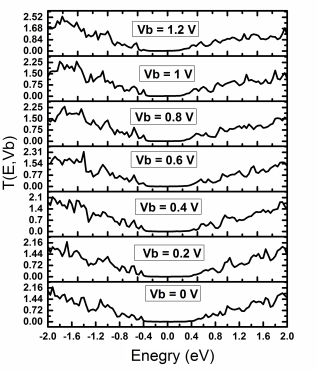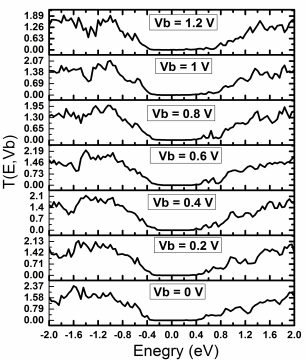with the HOMO of right electrode in spin-up channel because the bands in the two electrodes are shifted. These resonance states significantly enhance as bias increasing, which result in the rapid increase about I↑ after the bias of 0.75 V. Consequently, the rapid increase of I↑ also leads to the rapid decrease about η for PC setup after 0.75 V, already discussed above for Fig. 2(c).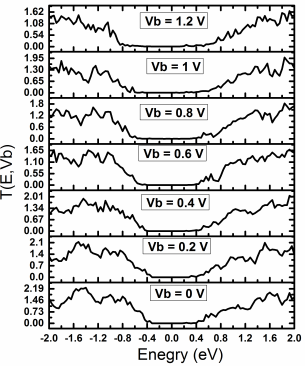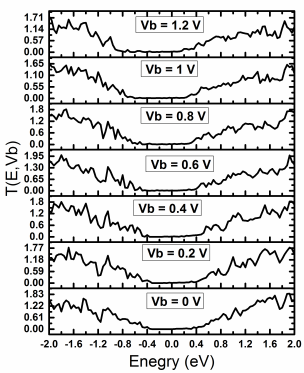The bias dependent T (E, Vb) for APC setup are more complex than PC setup. From Fig. 3(c), one can see that the T (E, Vb) in spin-up channel roughly maintains its shape when bias increases. A little resonance states A2 below Fermi level, which presents for the bias of 0.00 and 0.15 V are removed after the bias of 0.15 V. As the bias increase, only a little resonance states above the Fermi level enter to the transport window (i.e., the part of transmission function in the bias window integrated to obtain the current) and contribute to the I↑, which result in very slow increase of the I↑. After the bias of 0.15 V, the T (E, Vb) shifts to larger energy and large resonance states are induced around Fermi level due to the alignment of spin-down states around Fermi level of left electrode with HOMO of right electrode in spin-down channel because the bands in the two electrodes are shifted. These induced resonance states continuously enhance as bias increase, which result in rapid increase of I↓. Eventually, the total current for APC setup exceed PC setup after the bias of 0.75 V, result in negative RTMR, already discussed above for Fig. 2(c). As mentioned above, the quantum size effect leads to the quantized subbands in the cross section of 1D electrode. Hence, there are two peaks in the induced resonance states around Fermi level after the bias of 0.35 V, showed in Fig. 3(d). When T (E, Vb) continuously shifts to higher energies, these two peaks pass the Fermi level one by one, which cause the oscillation about the spin-down conductance. Thus, the spin injection factor η is oscillating with bias in the APC setup.

## IV. conclusion

We theoretically investigate the spin-polarized transport properties of V atoms doped (6,0) single-wall BNNT using non-equilibrium Green’s-function formalism with a self-consistent density- functional theory. The RTMR is as high as 789% at the bias of 0.05 V. It decays to zero and eventually to negative values when bias is increased. The ratio of spin injection is not below 99% until the bias of 0.55 V when the magnetic configurations of two electrodes are parallel. When the magnetic configurations of two electrodes are antiparallel, the ratio of spin injection oscillates with the bias. Our calculations indicate that it could design perfect 1D MTJs and spin filter using TMs doped BNNTs. The perfect TMR effect and spin filter effect are obtained.

## References

The template will number citations consecutively within brackets . The sentence punctuation follows the bracket . Refer simply to the reference number, as in —do not use “Ref. ” or “reference ” except at the beginning of a sentence: “Reference  was the first …”

Number footnotes separately in superscripts. Place the actual footnote at the bottom of the column in which it was cited. Do not put footnotes in the reference list. Use letters for table footnotes.

Unless there are six authors or more give all authors’ names; do not use “et al.”. Papers that have not been published, even if they have been submitted for publication, should be cited as “unpublished” . Papers that have been accepted for publication should be cited as “in press” . Capitalize only the first word in a paper title, except for proper nouns and element symbols.

For papers published in translation journals, please give the English citation first, followed by the original foreign-language citation .

1. G. Eason, B. Noble, and I.N. Sneddon, “On certain integrals of Lipschitz-Hankel type involving products of Bessel functions,” Phil. Trans. Roy. Soc. London, vol. A247, pp. 529-551, April 1955. (references)
2. J. Clerk Maxwell, A Treatise on Electricity and Magnetism, 3rd ed., vol. 2. Oxford: Clarendon, 1892, pp.68-73.
3. I.S. Jacobs and C.P. Bean, “Fine particles, thin films and exchange anisotropy,” in Magnetism, vol. III, G.T. Rado and H. Suhl, Eds. New York: Academic, 1963, pp. 271-350.
4. K. Elissa, “Title of paper if known,” unpublished.
5. R. Nicole, “Title of paper with only first word capitalized,” J. Name Stand. Abbrev., in press.
6. Y. Yorozu, M. Hirano, K. Oka, and Y. Tagawa, “Electron spectroscopy studies on magneto-optical media and plastic substrate interface,” IEEE Transl. J. Magn. Japan, vol. 2, pp. 740-741, August 1987 [Digests 9th Annual Conf. Magnetics Japan, p. 301, 1982].
7. M. Young, The Technical Writer’s Handbook. Mill Valley, CA: University Science, 1989.

View all

## DMCA / Removal Request

If you are the original writer of this essay and no longer wish to have your work published on UKEssays.com then please:

Related Services

Prices from

\$USD152

Approximate costs for:

• 1000 words
• 7 day deliveryHumanity University

Dedicated to your worth and value as a human being!

Related Lectures